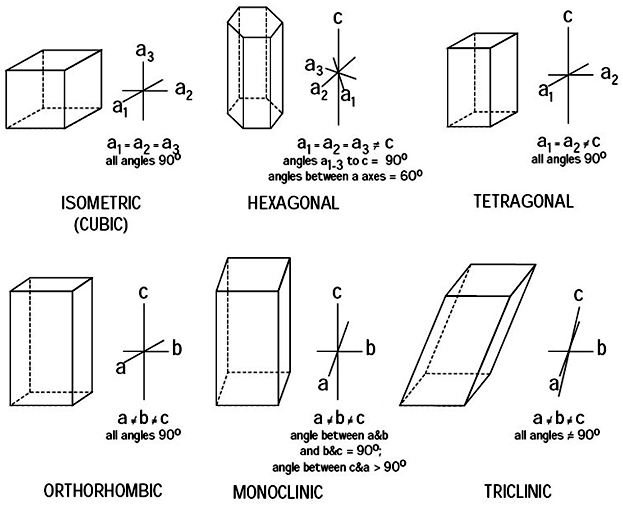Six types of Crystal Systems - Global Epidemic Observational Studies Organization

# Six types of Crystal Systems

There are hundreds of minerals on the earth, which are found in different crystal forms. These crystal forms are grouped into six major crystal forms. These crystal forms are as follows.### (1). Cubic or Isometric Crystal System:

List of ContentsThe crystals belonging to this system have three mutually perpendicular axes of equal lengths. These axes are designated as a1, a2, and a3. The axes of cubic/isometric crystal systems are as follows.

Read in detail; Cubic/Isometeric Crystal System

### (2). Tetragonal System of Crystals:

The crystals of this system are referred to as three mutually perpendicular axes. The two horizontal axes are equal (a1 and a2) and the vertical axis is longer or shorter than the other two.### (3). Hexagonal Crystal Systems:

In the crystals of the hexagonal system, there are four crystallographic axes. Three of these are of equal length and lie at angle of 120 degree to each other in the horizontal plane. These are designated as a1, a2, and a3. The fourth axis is vertical and is either longer or shorter than the other axes.

### (4). Orthorhombic Crystal Systems:

The crystals of this system have three mutually perpendicular axes of different lengths. They are designated as a, b, and c.

### (5). Monoclinic Crystal Systems:

In the crystals of this system, there are three unequal crystallographic axes. The ‘a’ and ‘c’ axes are inclined to each other at an oblique angle and the ‘b’ axis is perpendicular to the plane of the two.### (6). Axes of Triclinic Crystal System:

There are three unequal axes all intersecting at oblique angles.Related Articles: